# Convergence And Uniformity¶

## Introduction¶

Some parallel environments execute threads in groups that allow communication within the group using special primitives called convergent operations. The outcome of a convergent operation is sensitive to the set of threads that executes it “together”, i.e., convergently.

A value is said to be uniform across a set of threads if it is the same across those threads, and divergent otherwise. Correspondingly, a branch is said to be a uniform branch if its condition is uniform, and it is a divergent branch otherwise.

Whether threads are converged or not depends on the paths they take through the control flow graph. Threads take different outgoing edges at a divergent branch. Divergent branches constrain program transforms such as changing the CFG or moving a convergent operation to a different point of the CFG. Performing these transformations across a divergent branch can change the sets of threads that execute convergent operations convergently. While these constraints are out of scope for this document, the described uniformity analysis allows these transformations to identify uniform branches where these constraints do not hold.

Convergence and uniformity are inter-dependent: When threads diverge at a divergent branch, they may later reconverge at a common program point. Subsequent operations are performed convergently, but the inputs may be non-uniform, thus producing divergent outputs.

Uniformity is also useful by itself on targets that execute threads in groups with shared execution resources (e.g. waves, warps, or subgroups):

• Uniform outputs can potentially be computed or stored on shared resources.
• These targets must “linearize” a divergent branch to ensure that each side of the branch is followed by the corresponding threads in the same group. But linearization is unnecessary at uniform branches, since the whole group of threads follows either one side of the branch or the other.

This document presents a definition of convergence that is reasonable for real targets and is compatible with the currently implicit semantics of convergent operations in LLVM IR. This is accompanied by a uniformity analysis that extends the existing divergence analysis [DivergenceSPMD] to cover irreducible control-flow.

 [DivergenceSPMD] Julian Rosemann, Simon Moll, and Sebastian Hack. 2021. An Abstract Interpretation for SPMD Divergence on Reducible Control Flow Graphs. Proc. ACM Program. Lang. 5, POPL, Article 31 (January 2021), 35 pages. https://doi.org/10.1145/3434312

## Terminology¶

Cycles
Described in LLVM Cycle Terminology.
Closed path
Described in Closed Paths and Cycles.
Disjoint paths
Two paths in a CFG are said to be disjoint if the only nodes common to both are the start node or the end node, or both.
Join node
A join node of a branch is a node reachable along disjoint paths starting from that branch.
Diverged path
A diverged path is a path that starts from a divergent branch and either reaches a join node of the branch or reaches the end of the function without passing through any join node of the branch.

Each occurrence of an instruction in the program source is called a static instance. When a thread executes a program, each execution of a static instance produces a distinct dynamic instance of that instruction.

Each thread produces a unique sequence of dynamic instances:

• The sequence is generated along branch decisions and loop traversals.
• Starts with a dynamic instance of a “first” instruction.
• Continues with dynamic instances of successive “next” instructions.

Threads are independent; some targets may choose to execute them in groups in order to share resources when possible.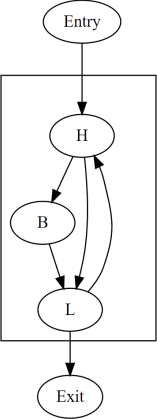1 2 3 4 5 6 7 8 9 Thread 1 Entry1 H1 B1 L1 H3 L3 Exit Thread 2 Entry1 H2 L2 H4 B2 L4 H5 B3 L5 Exit

In the above table, each row is a different thread, listing the dynamic instances produced by that thread from left to right. Each thread executes the same program that starts with an `Entry` node and ends with an `Exit` node, but different threads may take different paths through the control flow of the program. The columns are numbered merely for convenience, and empty cells have no special meaning. Dynamic instances listed in the same column are converged.

## Convergence¶

Converged-with is a transitive symmetric relation over dynamic instances produced by different threads for the same static instance. Informally, two threads that produce converged dynamic instances are said to be converged, and they are said to execute that static instance convergently, at that point in the execution.

Convergence order is a strict partial order over dynamic instances that is defined as the transitive closure of:

1. If dynamic instance `P` is executed strictly before `Q` in the same thread, then `P` is convergence-before `Q`.
2. If dynamic instance `P` is executed strictly before `Q1` in the same thread, and `Q1` is converged-with `Q2`, then `P` is convergence-before `Q2`.
3. If dynamic instance `P1` is converged-with `P2`, and `P2` is executed strictly before `Q` in the same thread, then `P1` is convergence-before `Q`.
 1 2 3 4 5 6 7 8 9 Thread 1 Entry … S2 T … Exit Thread 2 Entry … Q2 R S1 … Exit Thread 3 Entry … P Q1 …

The above table shows partial sequences of dynamic instances from different threads. Dynamic instances in the same column are assumed to be converged (i.e., related to each other in the converged-with relation). The resulting convergence order includes the edges ```P -> Q2```, `Q1 -> R`, `P -> R`, `P -> T`, etc.

The fact that convergence-before is a strict partial order is a constraint on the converged-with relation. It is trivially satisfied if different dynamic instances are never converged. It is also trivially satisfied for all known implementations for which convergence plays some role. Aside from the strict partial convergence order, there are currently no additional constraints on the converged-with relation imposed in LLVM IR.

Note

1. The `convergent` attribute on convergent operations does constrain changes to `converged-with`, but it is expressed in terms of control flow and does not explicitly deal with thread convergence.
2. The convergence-before relation is not directly observable. Program transforms are in general free to change the order of instructions, even though that obviously changes the convergence-before relation.
3. Converged dynamic instances need not be executed at the same time or even on the same resource. Converged dynamic instances of a convergent operation may appear to do so but that is an implementation detail. The fact that `P` is convergence-before `Q` does not automatically imply that `P` happens-before `Q` in a memory model sense.
4. Future work: Providing convergence-related guarantees to compiler frontends enables some powerful optimization techniques that can be used by programmers or by high-level program transforms. Constraints on the `converged-with` relation may be added eventually as part of the definition of LLVM IR, so that guarantees can be made that frontends can rely on. For a proposal on how this might work, see D85603.

### Maximal Convergence¶

This section defines a constraint that may be used to produce a maximal converged-with relation without violating the strict convergence-before order. This maximal converged-with relation is reasonable for real targets and is compatible with convergent operations.

The maximal converged-with relation is defined in terms of cycle headers, which are not unique to a given CFG. Each cycle hierarchy for the same CFG results in a different maximal converged-with relation.

Maximal converged-with:

Dynamic instances `X1` and `X2` produced by different threads for the same static instance `X` are converged in the maximal converged-with relation if and only if for every cycle `C` with header `H` that contains `X`:

• every dynamic instance `H1` of `H` that precedes `X1` in the respective thread is convergence-before `X2`, and,
• every dynamic instance `H2` of `H` that precedes `X2` in the respective thread is convergence-before `X1`,
• without assuming that `X1` is converged with `X2`.

Note

For brevity, the rest of the document restricts the term converged to mean “related under the maximal converged-with relation for the given cycle hierarchy”.

Maximal convergence can now be demonstrated in the earlier example as follows:

 1 2 3 4 5 6 7 8 9 Thread 1 Entry1 H1 B1 L1 H3 L3 Exit Thread 2 Entry2 H2 L2 H4 B2 L4 H5 B3 L5 Exit
• `Entry1` and `Entry2` are converged.
• `H1` and `H2` are converged.
• `B1` and `B2` are not converged due to `H4` which is not convergence-before `B1`.
• `H3` and `H4` are converged.
• `H3` is not converged with `H5` due to `H4` which is not convergence-before `H3`.
• `L1` and `L2` are converged.
• `L3` and `L4` are converged.
• `L3` is not converged with `L5` due to `H5` which is not convergence-before `L3`.

Contradictions in convergence order are possible only between two nodes that are inside some cycle. The dynamic instances of such nodes may be interleaved in the same thread, and this interleaving may be different for different threads.

When a thread executes a node `X` once and then executes it again, it must have followed a closed path in the CFG that includes `X`. Such a path must pass through the header of at least one cycle — the smallest cycle that includes the entire closed path. In a given thread, two dynamic instances of `X` are either separated by the execution of at least one cycle header, or `X` itself is a cycle header.

In reducible cycles (natural loops), each execution of the header is equivalent to the start of a new iteration of the cycle. But this analogy breaks down in the presence of explicit constraints on the converged-with relation, such as those described in future work. Instead, cycle headers should be treated as implicit points of convergence in a maximal converged-with relation.

Consider a sequence of nested cycles `C1`, `C2`, …, `Ck` such that `C1` is the outermost cycle and `Ck` is the innermost cycle, with headers `H1`, `H2`, …, `Hk` respectively. When a thread enters the cycle `Ck`, any of the following is possible:

1. The thread directly entered cycle `Ck` without having executed any of the headers `H1` to `Hk`.
2. The thread executed some or all of the nested headers one or more times.

The maximal converged-with relation captures the following intuition about cycles:

1. When two threads enter a top-level cycle `C1`, they execute converged dynamic instances of every node that is a child of `C1`.

2. When two threads enter a nested cycle `Ck`, they execute converged dynamic instances of every node that is a child of `Ck`, until either thread exits `Ck`, if and only if they executed converged dynamic instances of the last nested header that either thread encountered.

Note that when a thread exits a nested cycle `Ck`, it must follow a closed path outside `Ck` to reenter it. This requires executing the header of some outer cycle, as described earlier.

Consider two dynamic instances `X1` and `X2` produced by threads `T1` and `T2` for a node `X` that is a child of nested cycle `Ck`. Maximal convergence relates `X1` and `X2` as follows:

1. If neither thread executed any header from `H1` to `Hk`, then `X1` and `X2` are converged.
2. Otherwise, if there are no converged dynamic instances `Q1` and `Q2` of any header `Q` from `H1` to `Hk` (where `Q` is possibly the same as `X`), such that `Q1` precedes `X1` and `Q2` precedes `X2` in the respective threads, then `X1` and `X2` are not converged.
3. Otherwise, consider the pair `Q1` and `Q2` of converged dynamic instances of a header `Q` from `H1` to `Hk` that occur most recently before `X1` and `X2` in the respective threads. Then `X1` and `X2` are converged if and only if there is no dynamic instance of any header from `H1` to `Hk` that occurs between `Q1` and `X1` in thread `T1`, or between `Q2` and `X2` in thread `T2`. In other words, `Q1` and `Q2` represent the last point of convergence, with no other header being executed before executing `X`.

Example: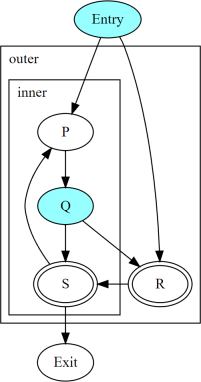The above figure shows two nested irreducible cycles with headers `R` and `S`. The nodes `Entry` and `Q` have divergent branches. The table below shows the convergence between three threads taking different paths through the CFG. Dynamic instances listed in the same column are converged.

 1 2 3 4 5 6 7 8 10 Thread1 Entry P1 Q1 S1 P3 Q3 R1 S2 Exit Thread2 Entry P2 Q2 R2 S3 Exit Thread3 Entry R3 S4 Exit
• `P2` and `P3` are not converged due to `S1`
• `Q2` and `Q3` are not converged due to `S1`
• `S1` and `S3` are not converged due to `R2`
• `S1` and `S4` are not converged due to `R3`

Informally, `T1` and `T2` execute the inner cycle a different number of times, without executing the header of the outer cycle. All threads converge in the outer cycle when they first execute the header of the outer cycle.

## Uniformity¶

1. The output of two converged dynamic instances is uniform if and only if it compares equal for those two dynamic instances.
2. The output of a static instance `X` is uniform for a given set of threads if and only if it is uniform for every pair of converged dynamic instances of `X` produced by those threads.

A non-uniform value is said to be divergent.

For a set `S` of threads, the uniformity of each output of a static instance is determined as follows:

1. The semantics of the instruction may specify the output to be uniform.
2. Otherwise, if it is a PHI node, its output is uniform if and only if for every pair of converged dynamic instances produced by all threads in `S`:
1. Both instances choose the same output from converged dynamic instances, and,
2. That output is uniform for all threads in `S`.
3. Otherwise, the output is uniform if and only if the input operands are uniform for all threads in `S`.

### Divergent Cycle Exits¶

When a divergent branch occurs inside a cycle, it is possible that a diverged path continues to an exit of the cycle. This is called a divergent cycle exit. If the cycle is irreducible, the diverged path may re-enter and eventually reach a join within the cycle. Such a join should be examined for the diverged entry criterion.

Nodes along the diverged path that lie outside the cycle experience temporal divergence, when two threads executing convergently inside the cycle produce uniform values, but exit the cycle along the same divergent path after executing the header a different number of times (informally, on different iterations of the cycle). For a node `N` inside the cycle the outputs may be uniform for the two threads, but any use `U` outside the cycle receives a value from non-converged dynamic instances of `N`. An output of `U` may be divergent, depending on the semantics of the instruction.

## Static Uniformity Analysis¶

Irreducible control flow results in different cycle hierarchies depending on the choice of headers during depth-first traversal. As a result, a static analysis cannot always determine the convergence of nodes in irreducible cycles, and any uniformity analysis is limited to those static instances whose convergence is independent of the cycle hierarchy:

m-converged static instances:

A static instance `X` is m-converged for a given CFG if and only if the maximal converged-with relation for its dynamic instances is the same in every cycle hierarchy that can be constructed for that CFG.

Note

In other words, two dynamic instances `X1` and `X2` of an m-converged static instance `X` are converged in some cycle hierarchy if and only if they are also converged in every other cycle hierarchy for the same CFG.

As noted earlier, for brevity, we restrict the term converged to mean “related under the maximal converged-with relation for a given cycle hierarchy”.

Each node `X` in a given CFG is reported to be m-converged if and only if:

1. `X` is a top-level node, in which case, there are no cycle headers to influence the convergence of `X`.
2. Otherwise, if `X` is inside a cycle, then every cycle that contains `X` satisfies the following necessary conditions:
1. Every divergent branch inside the cycle satisfies the diverged entry criterion, and,
2. There are no diverged paths reaching the cycle from a divergent branch outside it.

Note

A reducible cycle trivially satisfies the above conditions. In particular, if the whole CFG is reducible, then all nodes in the CFG are m-converged.

If a static instance is not m-converged, then every output is assumed to be divergent. Otherwise, for an m-converged static instance, the uniformity of each output is determined using the criteria described earlier. The discovery of divergent outputs may cause their uses (including branches) to also become divergent. The analysis propagates this divergence until a fixed point is reached.

The convergence inferred using these criteria is a safe subset of the maximal converged-with relation for any cycle hierarchy. In particular, it is sufficient to determine if a static instance is m-converged for a given cycle hierarchy `T`, even if that fact is not detected when examining some other cycle hierarchy `T'`.

This property allows compiler transforms to use the uniformity analysis without being affected by DFS choices made in the underlying cycle analysis. When two transforms use different instances of the uniformity analysis for the same CFG, a “divergent value” result in one analysis instance cannot contradict a “uniform value” result in the other.

Generic transforms such as SimplifyCFG, CSE, and loop transforms commonly change the program in ways that change the maximal converged-with relations. This also means that a value that was previously uniform can become divergent after such a transform. Uniformity has to be recomputed after such transforms.

### Divergent Branch inside a Cycle¶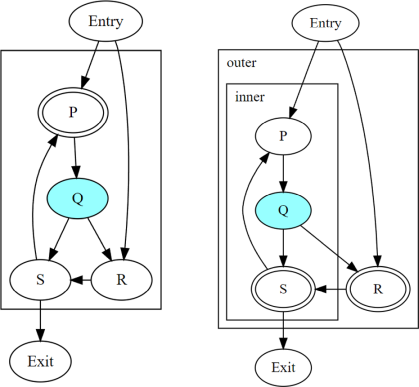The above figure shows a divergent branch `Q` inside an irreducible cyclic region. When two threads diverge at `Q`, the convergence of dynamic instances within the cyclic region depends on the cycle hierarchy chosen:

1. In an implementation that detects a single cycle `C` with header `P`, convergence inside the cycle is determined by `P`.
2. In an implementation that detects two nested cycles with headers `R` and `S`, convergence inside those cycles is determined by their respective headers.

A conservative approach would be to simply report all nodes inside irreducible cycles as having divergent outputs. But it is desirable to recognize m-converged nodes in the CFG in order to maximize uniformity. This section describes one such pattern of nodes derived from closed paths, which are a property of the CFG and do not depend on the cycle hierarchy.

Diverged Entry Criterion:

The dynamic instances of all the nodes in a closed path `P` are m-converged only if for every divergent branch `B` and its join node `J` that lie on `P`, there is no entry to `P` which lies on a diverged path from `B` to `J`.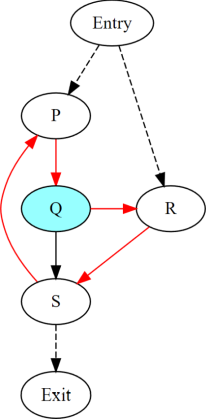Consider the closed path `P -> Q -> R -> S` in the above figure. `P` and `R` are entries to the closed path. `Q` is a divergent branch and `S` is a join for that branch, with diverged paths `Q -> R -> S` and ```Q -> S```.

• If a diverged entry `R` exists, then in some cycle hierarchy, `R` is the header of the smallest cycle `C` containing the closed path and a child cycle `C'` exists in the set `C - R`, containing both branch `Q` and join `S`. When threads diverge at `Q`, one subset `M` continues inside cycle `C'`, while the complement `N` exits `C'` and reaches `R`. Dynamic instances of `S` executed by threads in set `M` are not converged with those executed in set `N` due to the presence of `R`. Informally, threads that diverge at `Q` reconverge in the same iteration of the outer cycle `C`, but they may have executed the inner cycle `C'` differently.

 1 2 3 4 5 6 7 8 9 10 11 Thread1 Entry P1 Q1 R1 S1 P3 … Exit Thread2 Entry P2 Q2 S2 P4 Q4 R2 S4 Exit

In the table above, `S2` is not converged with `S1` due to `R1`.

• If `R` does not exist, or if any node other than `R` is the header of `C`, then no such child cycle `C'` is detected. Threads that diverge at `Q` execute converged dynamic instances of `S` since they do not encounter the cycle header on any path from `Q` to `S`. Informally, threads that diverge at `Q` reconverge at `S` in the same iteration of `C`.

 1 2 3 4 5 6 7 8 9 10 Thread1 Entry P1 Q1 R1 S1 P3 Q3 R3 S3 Exit Thread2 Entry P2 Q2 S2 P4 Q4 R2 S4 Exit

Note

In general, the cycle `C` in the above statements is not expected to be the same cycle for different headers. Cycles and their headers are tightly coupled; for different headers in the same outermost cycle, the child cycles detected may be different. The property relevant to the above examples is that for every closed path, there is a cycle `C` that contains the path and whose header is on that path.

The diverged entry criterion must be checked for every closed path passing through a divergent branch `B` and its join `J`. Since every closed path passes through the header of some cycle, this amounts to checking every cycle `C` that contains `B` and `J`. When the header of `C` dominates the join `J`, there can be no entry to any path from the header to `J`, which includes any diverged path from `B` to `J`. This is also true for any closed paths passing through the header of an outer cycle that contains `C`.

Thus, the diverged entry criterion can be conservatively simplified as follows:

For a divergent branch `B` and its join node `J`, the nodes in a cycle `C` that contains both `B` and `J` are m-converged only if:

• `B` strictly dominates `J`, or,
• The header `H` of `C` strictly dominates `J`, or,
• Recursively, there is cycle `C'` inside `C` that satisfies the same condition.

When `J` is the same as `H` or `B`, the trivial dominance is insufficient to make any statement about entries to diverged paths.

### Diverged Paths reaching a Cycle¶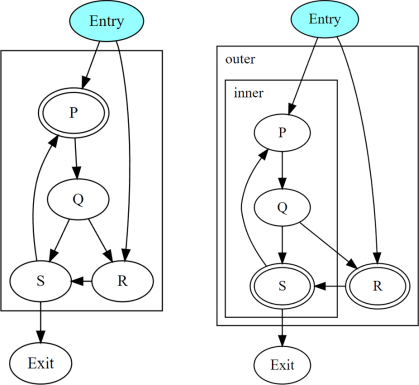The figure shows two cycle hierarchies with a divergent branch in `Entry` instead of `Q`. For two threads that enter the closed path `P -> Q -> R -> S` at `P` and `R` respectively, the convergence of dynamic instances generated along the path depends on whether `P` or `R` is the header.

• Convergence when `P` is the header.

 1 2 3 4 5 6 7 8 9 10 11 12 13 Thread1 Entry P1 Q1 R1 S1 P3 Q3 S3 Exit Thread2 Entry R2 S2 P2 Q2 S2 P4 Q4 R3 S4 Exit

• Convergence when `R` is the header.

 1 2 3 4 5 6 7 8 9 10 11 12 Thread1 Entry P1 Q1 R1 S1 P3 Q3 S3 Exit Thread2 Entry R2 S2 P2 Q2 S2 P4 … Exit

Thus, when diverged paths reach different entries of an irreducible cycle from outside the cycle, the static analysis conservatively reports every node in the cycle as not m-converged.

### Reducible Cycle¶

If `C` is a reducible cycle with header `H`, then in any DFS, `H` must be the header of some cycle `C'` that contains `C`. Independent of the DFS, there is no entry to the subgraph `C` other than `H` itself. Thus, we have the following:

1. The diverged entry criterion is trivially satisfied for a divergent branch and its join, where both are inside subgraph `C`.
2. When diverged paths reach the subgraph `C` from outside, their convergence is always determined by the same header `H`.

Clearly, this can be determined only in a cycle hierarchy `T` where `C` is detected as a reducible cycle. No such conclusion can be made in a different cycle hierarchy `T'` where `C` is part of a larger cycle `C'` with the same header, but this does not contradict the conclusion in `T`.